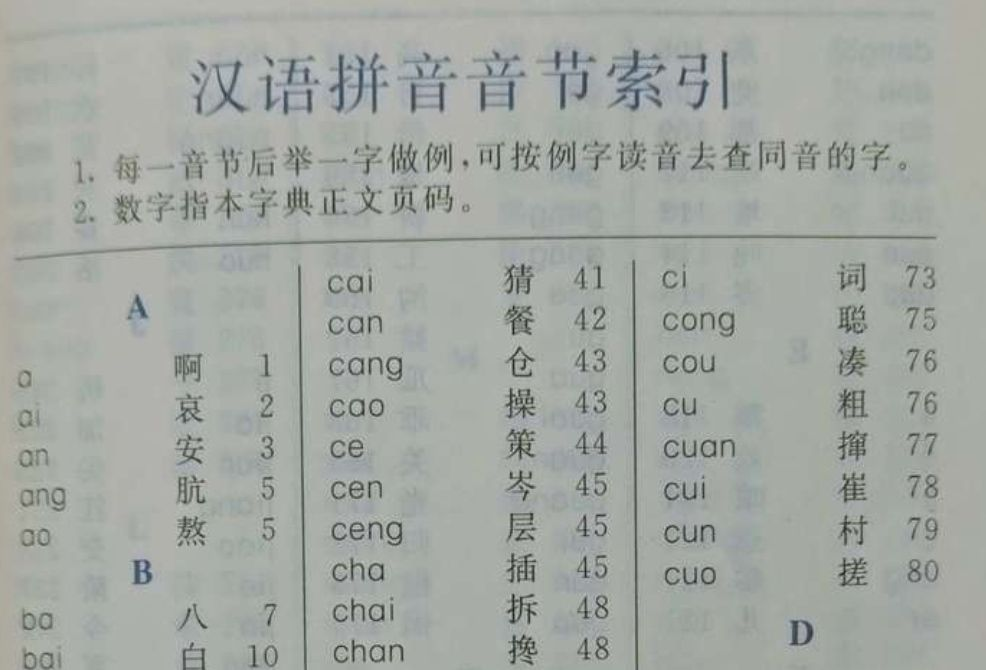# python字典(dict)详解

## 1. 字典的定义

python的字典(dict)属于映射类型，是数据的无序集合，字典内的元素都是key:value形式的键值对，所有键值对存储在一对大花括号{}中，每一个键值对之间使用逗号分隔。

``````{'name': '小明', 'age': 14}
``````

``````int_dict = {
1: '1做key',
True: 'True做key'
}

print(int_dict)         # {1: 'True做key'}
``````

## 2. 创建python字典

### 2.1 使用{}创建字典

``````empty_dict = {}
``````

``````contacts_dict = {
"小王": '13892339876',
"小张": '13898320987',
"小李": '13890348745'
}
``````

``````print(len(contacts_dict))   # 3
``````

### 2.2 使用内置函数dict创建字典

dict函数的语法如下

``````class dict(**kwarg)
class dict(mapping, **kwarg)
class dict(iterable, **kwarg)
``````

• kwarg 关键字参数
• mapping 关联式的容器类型
• iterable 可迭代对象

dict函数返回一个新的字典，下面是使用示例

``````>>> new_dict = dict({'name': '小明', 'age': 14})
>>> new_dict
{'name': '小明', 'age': 14}
``````

``````>>> new_dict = dict(name='小明', age=14)
>>> new_dict
{'name': '小明', 'age': 14}
``````

``````>>> new_dict = dict([('name', '小明'), ('age', 14)])
>>> new_dict
{'name': '小明', 'age': 14}
``````

## 3. 字典新增键值对

``````score_dict = {
'小明': 96,
'小刚': 98,
'小红': 94
}

score_dict['小丽'] = 100
``````

## 4. 修改字典

``````score_dict = {
'小明': 96,
'小刚': 98,
'小红': 94
}
score_dict['小明'] = 100
print(score_dict)
``````

``````{
'小明': 100,
'小刚': 98,
'小红': 94
}
``````

``````dict[key] = value
``````

## 5. 删除字典里的元素

``````score_dict = {
'小明': 96,
'小刚': 98,
'小红': 94
}

del score_dict['小明']
print(score_dict)           # {'小刚': 98, '小红': 94}
``````

``````score_dict = {
'小明': 96,
'小刚': 98,
'小红': 94
}

score_dict.pop('小明')
print(score_dict)           # {'小刚': 98, '小红': 94}
``````

``````score_dict = {
'小明': 96,
'小刚': 98,
'小红': 94
}

score_dict.clear()
print(score_dict)           # {}
``````

## 6. 获取字典里的值``````score_dict = {
'小明': 99,
'小刚': 98,
'小红': 94
}

print(score_dict['小红'])       # 94
``````

``````print(score_dict['小丽'])
``````

``````Traceback (most recent call last):
File "/Users/kwsy/PycharmProjects/pythonclass/mytest/demo.py", line 7, in <module>
print(score_dict['小丽'])
KeyError: '小丽'
``````

KeyError表示不存在'小丽'这个key

## 7 遍历字典

``````score_dict = {
'小明': 96,
'小刚': 98,
'小红': 94
}

for key in score_dict:
print(key, score_dict[key])
``````

``````score_dict = {
'小明': 96,
'小刚': 98,
'小红': 94
}

for key, value in score_dict.items():
print(key, value)
``````

``````小明 96

``````

## 8. 字典常用方法

clear() 删除字典内所有的元素
copy() 返回字典的浅复制
fromkeys() 以指定key创建一个新的字典
get() 返回指定key的值
items() 成对返回所有key和value
keys() 返回字典所有的key
values 返回字典所有value
setdefault() 为key设置对应的默认值
update() 更新字典
pop() 删除键值对QQ交流群: 211426309